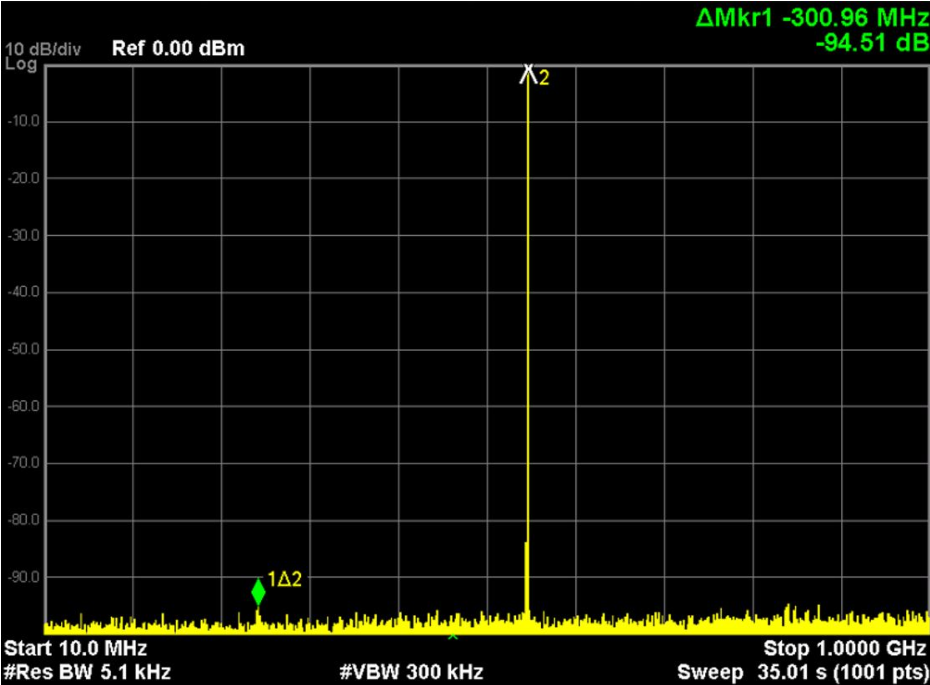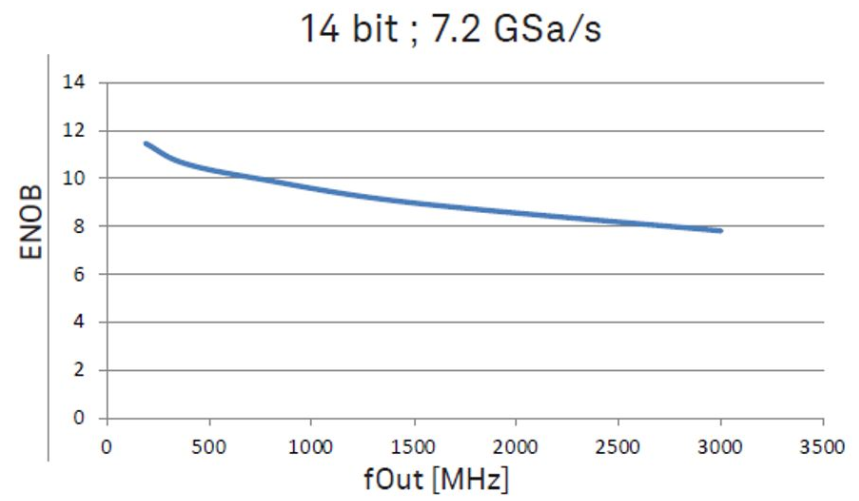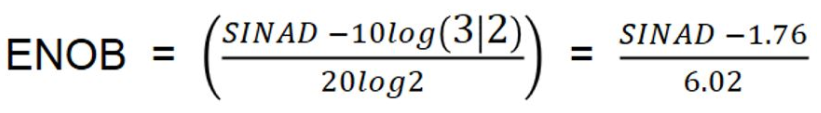save Save

# Know Your Top Arbitrary Waveform Generator Specifications

### Summary

Knowing key Arbitrary Waveform Generator (AWG) specifications lets you make the best purchase decision, saving you time and money. Learn how to compare these characteristics across arbitrary waveform generator types and vendors: memory, sample rate, dynamic range, and bandwidth.

# Know Your Top Arbitrary Waveform Generator Specifications

Knowing key Arbitrary Waveform Generator (AWG) specifications lets you make the best purchase decision, saving you time and money. Learn how to compare these characteristics across arbitrary waveform generator types and vendors: memory, sample rate, dynamic range, and bandwidth. Let’s discuss these specifications in detail.

Memory Size

Memory size is the amount of memory available for storing long strings of user-defined waveforms. This specification is listed in giga samples (GSa). Data is fed into the digital-to-analog converter (DAC), which creates a voltage stair-stepped representation of your desired signal. High sample rates and large memories are needed to accurately create the defined signal.

Sample Rate

Sample rate is the number of samples the DAC can take in a given time interval. The specification is listed in giga samples per second (GSa/s). The sample rate determines the maximum frequency component of the arbitrary waveform generator output signal. Other industry terms for sample rate are “clock rate” and “sample access rate.” A key formula to understand the relationship between memory size and sample rate is shown below.

Memory / sample rate = play time

You can see from the formula above that as the sample rate increases, more memory is used and play time decreases. Play time determines the total length of the unique waveform an arbitrary waveform generator can generate. This play time length is also called time before repeating. For example, a memory size of 256 kSa and a sample rate of 64 GSa/s results in a play time of 4 micro seconds. This is not a very long pattern and is why large amounts of memory are needed for more play time.

Dynamic Range - Vertical Resolution (ADC Bits)

This is the output of the DAC, and it is expressed in voltage as vertical bits of resolution. The value is listed in decibels relative to carrier amplitude (dBc). For example, an 8-bit DAC can output two to the eighth bits of vertical resolution or 256 different voltage levels creating the desired waveform. When comparing arbitrary waveform generator ADC bits specification across different brands, it is important to know that for every ADC bit added, the vertical resolution is doubled.

Bandwidth

Arbitrary waveform generator outputs are limited to a specific upper-end output frequency. The bandwidth of the arbitrary waveform generator is the range of frequency outputs that it can reliably provide. The value is also called “data rate” and is listed in giga bits per second (Gb/s). Note that the bandwidth is determined by the sample rate, but it will not be a one-to-one correlation. Let’s walk through the reasons why this is the case:

1. The DAC must accurately create the signal in memory and needs to create at least two data points per period. This is called the Nyquist Theory. So, a sample rate of 1 GHz results in a DAC output of 500 MHz or one half the sample rate.
2. The DAC output signal is not a smooth sinewave but is a voltage stepped representation of the pattern in memory. Because of this, the DAC output needs to be filtered. Within the arbitrary waveform generator, this filtering is done by what is called a reconstruction filter, which creates a smooth sinewave. However, there is a price for this filtering in the form of an additional 10% loss to the sample-rate-to-AWG-output frequency ratio. You can reference the formula below. For example, the 1 GHz sample rate mentioned above results in an arbitrary waveform generator maximum output frequency of 400 MHz.

Maximum arbitrary waveform generator output frequency = sample rate x 40%

Spurious Free Dynamic Range (SFDR)

This is measured in the frequency domain and is the distance in dB from the selected frequency to the highest visible spur or harmonic within the stated bandwidth. The value is listed in decibels relative to the selected frequency amplitude. The screen shot in Figure 1 below shows an example of an AWG output frequency in the center of the screen with a spur to the left that is 94.54 dB lower than its amplitude.Figure 1. Center frequency relative to a spur.

Effective Number of Bits (ENOB)

The effective number of bits is derived from the DAC bits. It is a lesser value than the DAC bits due to impacts of harmonics, spurious signals, and the AWG noise floor. Note that this specification changes over the bandwidth of the arbitrary waveform generator, and you should look at ENOB vs. frequency plots like the one below to see the value associated with your signal out frequency of choice. Note the plot in Figure 2 below references a 14-bit system. But after the impacts of receiver signal to noise and distortion (SINAD), you can see that at 1.5 GHz, the actual bit of resolution is deceased to around 9 bits.Figure 2. An ENOB curve over frequency.

ENOB is a great specification to see the actual performance of the arbitrary waveform generator after factoring in these effects on signal quality. ENOB can be measured or calculated. (The formula is below.) Note that SINAD is the ratio of total signal power to unwanted signal noise.Jitter

A waveform’s jitter can cause misalignment of edges and voltage levels. This can cause the AWG to inject data errors into your system. The jitter value is usually listed in ps peak-peak between the sync clock and the direct data output.

Conclusion

Know your key arbitrary waveform generator specifications to properly select the AWG that fits your application:

• Memory, sample rate, and play time are interrelated.
• Your bandwidth will not match your sample rate but will be 60% of that number.
• ENOB is a better representation of resolution than ADC bits.
• Factor in the jitter specification to account for true signal fidelity